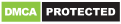# Math games: arithmetic, times tables, mental math

### Additional Information

Genres
Version
-
Developer
-
Requires
-
Updated
-Math has never been so easy. More than 2 000 000 of our users have mastered mental math tricks and times tables using our math games. Now is your turn to be a math master!

Unique functionality: listen 🔈 🎧 math exercises and solve math tasks by voice 🎙️in hands-free mode!

Our math trainer app has collected the most effective mental math tricks. It’s designed as an interactive tutorial with math games for your brain, where you get acquainted with each math method, and then practice it having different types of brain math workouts and math exercises. Study of mental arithmetic tricks will be an exciting brain training math games for you: solve tasks, get degrees, win stars and trophies.

The application “Math games: arithmetic, times tables, mental math” will be useful for all:
✓ kids – master the basics of arithmetic, learn multiplication table
✓ students – do everyday math practice, prepare for math exercises or examine
✓ adults – keep their mind and brain in good shape, improve results in iq test, quickly solve logic games

🎓 Learning:
More than 30 math tricks for all grades:
☆ 1st grade math: addition and subtraction of single digits
☆ 2nd grade math: addition and subtraction of double digits, multiplication of single digits (multiplication table)
☆ 3rd grade math: addition and subtraction of triple digits, multiplication and division of double digits
☆ 4th grade math: multiplication and division of triple digits, percentages, square root
☆ 5th, 6th, etc. we have mental math games for all grades and ages! Math practice is a key for success.

🏁 Training:
☆ train quality by passing a fast math workout to get bachelor’s, master’s or professor’s degree
☆ train speed by solving 10 math exercises as fast as you can to get copper, silver or gold cup
☆ train complexity by solving as much math tasks as you want with configurable complexity
☆ train result by solving as much math exercises as you can in 60 seconds (brainstorm)
☆ train endurance by solving as much arithmetic tasks as you want without time limits
☆ work on mistakes

❌ Times tables:
☆ basic times tables 2..9 x 2..9
☆ advanced times tables 2..19 x 2..19
☆ fully configurable fast math workout on complexity 1..999 x 1…999

⌚Smart watch:
☆ solve as much math tasks as you can in 60 seconds
☆ fully configurable math exercises complexity (addition, subtraction, multiplication, division)
☆ ability to use different task modes
☆ listen math task using smart watch speaker

Math can be fun. What are you waiting for? Download our free game “Math games: arithmetic, times tables, mental math” and discover math world today!
☆ minor improvements 🚀
☆ bug fixes 🍭

🇺🇸 Thank you for choosing us 🏆 If you are ready to help with app translation into other languages, just let us know mentalcalculationtricks@gmail.com Pro version for all translators!

Recommended for you

You may also like

Comments